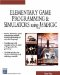# Random

This command generates a random number.

` randomnumber = random(value) `

### Parameters

 randomnumber A random number between 0 and value value Specifies what the maximum possible value is of the random number

### Notes

The random command is useful for making a game behave in a different way every time. For example, the position of an object at game start-up could be determined by a random number so that the location of the object will be different each time the game is played . The following code shows this happening.

The random number will generate the same random number each time unless you give it a different seed value. The SetRandom(System.GetElapsedTime()) command gives a seed value to the random number generator that is linked to the time. In this way, you will get a different set of random numbers each time.

### Sample Code

Please run the following code several times so that you can see the difference in how the sphere is placed.

` oworld = New World(); ocamera = New Camera(oworld); sphere = oworld.CreateSphere(50,50,50,25,25); ocamera.MoveBack(1200); sphere.Walk(); SetRandom(System.GetElapsedTime()); X = random(200); Sphere.SetPosition(x,0,0); SetText("random no " + x); //Loop While(TRUE)  {   } `Elementary Game Programming & Simulations Using Jamagic (Charles River Media Game Development)
ISBN: 1584502614
EAN: 2147483647
Year: 2002
Pages: 105
Authors: Sergio Perez